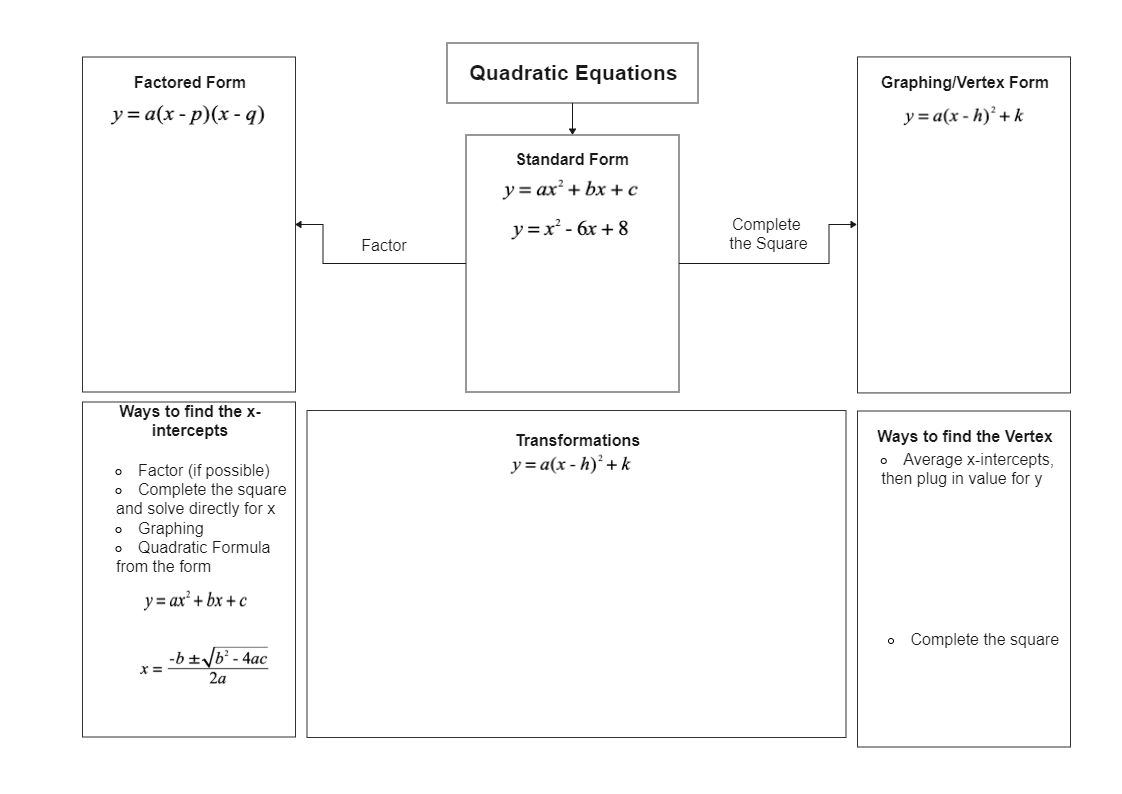Share
Duplicate3
0
Report
Publish time：11-08-2021
Quadratics forms is a mathematical concept. It is a polynomial. There are three forms of quadratic equations- Standard form, Factored form, and Vertex form. The origin of the Quadratic forms was first recorded in 1855-60. Although an Indian mathematician named Brahmagupta worked on similar equations in the year 628. In Europe, quadratic forms were mainly studied by Brounckner, Euler, and Lagrange. In the year 1801, Gauss wrote extensively on the theory of binary quadratic forms over the integers. Quadratic forms are polynomials but when there are one, two, or three variables these are called as unary, binary, and ternary. There are several methods of solving quadratic forms. Four of these methods are- factoring, using the square roots, quadratic formula, and completing the square.DANIELLE SCHIMSCHALPost
Recommended Templates